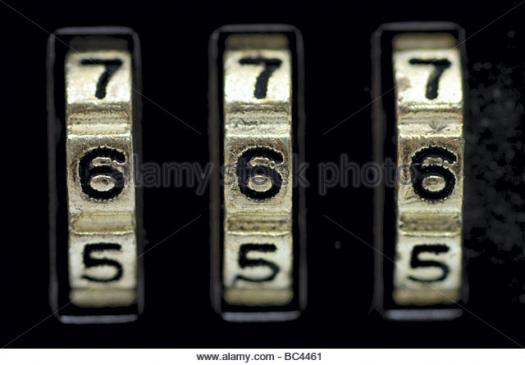# How Well Do You Know One-step Multiplication And Division Equations?

10 Questions | Total Attempts: 103SettingsCreate your own QuizHow well do you know one-step multiplication and division equations? In mathematics, an equation is a statement of an equality containing one or more variables. Solving the equation consists of determining which values of the variables make the equality true.Back to top